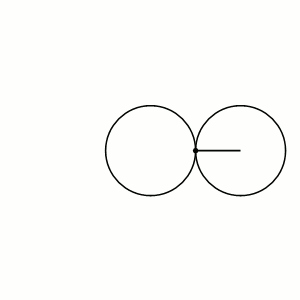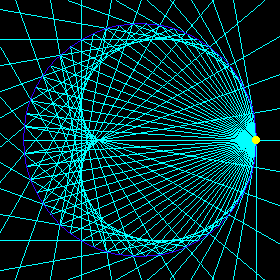## Rolling Circles and Balls (Part 1)

#### John Baez

For over a decade I've been struggling with certain math puzzle, first with the help of James Dolan and later also with John Huerta. It's about something amazing that happens when you roll a ball on another ball that's exactly 3 times as big. John Huerta and I just finished a paper about it, and I'd like to explain that here.

But I'd like to ease into it slowly, so I'll start by talking about what happens when you roll a circle on another circle that's the exact same size:Can you see how the rolling circle rotates twice as it rolls around the fixed circle once? Do you understand why?

The heart-shaped curve traced out by any point on the rolling circle is called a cardioid. In her latest video Vi Hart pretends to complain about parabolas while actually telling us quite a lot about them, and much else too:

Naturally, with her last name, she prefers the cardioid. She describes various ways to draw this curve: for example, by turning the hated parabola inside out. Here are my 6 favorite ways:

1) The one we've seen already: roll a circle on another circle the same size, and track the motion of a point on the rolling circle:2) Take a parabola and 'turn it inside out', replacing each point with polar coordinates $$(r, \theta)$$ by a point with coordinates $$(1/r, \theta)$$. As long as your parabola doesn't contain the origin, you get a cardioid:This 'turning inside out' trick is called conformal inversion.

3) Draw all circles whose centers are points on a fixed circle, and which contain a specified point on that circle:Here's a nice animation of this process, made available by the Math Images Project under a GNU Free Documentation License:

4) Let light rays emanate from one point on a circle and reflect off all other points on that circle. Draw all these reflected rays, and you'll see a cardioid:If you draw all light rays that reflect off some curve, the curve they snuggle up against (their so-called envelope) is called a catacaustic.

5) Draw 36 equally spaced points on a circle numbered 0 to 35, and draw a line between each point $$n$$ and the point $$2n$$ modulo 36. You'll see a cardioid, approximately:But there's nothing special about the number 36. If you take more evenly spaced points, you get a better approximation to a cardioid. You get a perfect cardioid if you connect each point $$(1, \theta)$$ to the point $$(1, 2\theta)$$ with a line, and take the envelope of these lines.

6) Finally, here's how to draw a cardioid starting with a cardioid! Draw all the osculating circles of the cardioid—that is, circles match the cardioid's curvature as well as its slope at the points they touch. The centers of these circles give another cardioid:This picture has some distracting lines on it; just look at the big and the little cardioid, and the circles. This trick is an example of an 'evolute'. The evolute of a curve is the set of centers of the osculating circles of that curve.

The last three pictures are from Xah Lee's wonderful website or the Wikipedia article on cardioids. Click on the picture to see where it came from and get more information.

I ❤ cardioids!

Next time we'll see what happens when we roll a circle inside a circle that's exactly twice as big.

### Constructions on curves

We've seen a few constructions on curves:

• A roulette is the curve traced out by a point attached to a given curve as it rolls without slipping along a second curve.

We rolled a circle on a circle and got a cardioid, but you could roll a parabola on another parabola:This gives a curve called the cissoid of Diocles, which in some coordinate system (not the one shown) is given by this cubic equation:

$$(x^2+y^2)x=2ay^2$$

• A catacaustic is the envelope of rays emanating from a specified point (perhaps a point at infinite distance, which produces parallel rays) and reflecting off a given curve.

We've obtained the cardioid as a catacaustic of the circle. Supposedly if you take the cissoid of Diocles and form its catacaustic using rays emanating from its 'focus', you get a cardioid! This would be a seventh way to get a cardioid, but I don't understand it, even though it's described on Wolfram Mathworld. I don't even know what the 'focus' of a cissoid of Diocles is. Can you help?

• The evolute of a curve is the curve formed by the centers of its tangent circles.

We've seen that the cardioid is its own evolute. The evolute of an ellipse looks like this:It's called an astroid, and it's given by an equation of this form:

$$a x^{2/3} + b y^{2/3} = 1$$

If you take the tangent circles of the black points on the ellipse above, their centers are the sharp pointy 'cusps' of the astroid.

### Order from chaos?

Some other famous ways to construct new curves from old ones include the involute, the isoptic, and the pedal. I could describe them... but I won't. You get the picture: there's a zoo of curves and constructions on curves, and lots of relations between these constructions. It's all very beautiful, but also a bit of a mess.

It seems that all these constructions, and their relations, should be studied more systematically in algebraic geometry. It may seem like a somewhat musty and old-fashioned branch of algebraic geometry, but surely there's a way to make it new and fresh using modern math. Has someone done this?